# Transformations in HTML5 Canvas

 SEARCH THIS SITE @import url(http://www.google.com/cse/api/branding.css);Custom Search
Some examples of transforming drawings and shapes in HTML5 canvas

## 1 - Translation

Basic transform methods may be used to translate, rotate and scale complicated shapes in an HTML 5 canvas. We first look at translating shapes.

Copy and paste the code below in Notepad++ (or any other HTML5 editor) and run it.

<!DOCTYPE html>
<html>
<body>
<canvas id="myFirstCanvas" width="500" height="401" style="border:4px solid #0000ff;">
This browser does not support the HTML5 canvas tag. </canvas>
<script>

var canvas1 = document.getElementById('myFirstCanvas');
var context1 = canvas1.getContext('2d');

// draw shape

context1.beginPath();
context1.strokeStyle = "green";
context1.lineWidth = 10;
context1.rect(100,100,150,100);
context1.stroke();

// draw same shape translated

context1.translate(100,150);

context1.beginPath();
context1.strokeStyle = "red";
context1.lineWidth = 10;
context1.rect(100,100,150,100);
context1.stroke();

</script>
</body>
</html>

Explanations
In this example, a rectangle is translated 100 pixels along the x-axis and 150 pixels along the y-axis using the method translate() in the statement context1.translate(100,150);.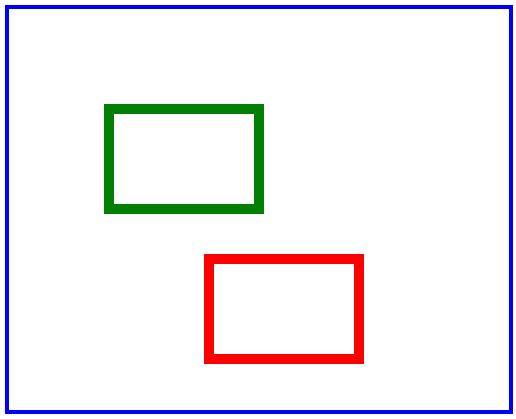.

## 2 - Rotation

We now look at rotating shapes with respect to the origin (0,0) of the canvas coordinate system (top left corner).

Copy and paste the code below in Notepad++ (or any other HTML5 editor) and run it.

<!DOCTYPE html>
<html>
<body>
<canvas id="myFirstCanvas" width="500" height="401" style="border:1px solid #0000ff;">
This browser does not support the HTML5 canvas tag. </canvas>

<script>

var canvas1 = document.getElementById('myFirstCanvas');
var context1 = canvas1.getContext('2d');

// Draw segment along x axis.

context1.beginPath();
context1.strokeStyle = "red";
context1.lineWidth = 3;
context1.moveTo(0,0);
context1.lineTo(300,0);
context1.stroke();

// Rotate segment clockwise by 45 degrees
context1.rotate((45)*Math.PI/180);

context1.beginPath();
context1.strokeStyle = "red";
context1.lineWidth = 3;
context1.moveTo(0,0);
context1.lineTo(300,0);
context1.stroke();

</script>
</body>
</html>

Explanations
A segment is rotated 45 degrees clockwise with respect to the origin (0,0) of the canvas. The method rotate() in the statement context1.rotate((45)*Math.PI/180); is used to rotate the segment 45 degrees converted to radians using the formula (45)*Math.PI/180.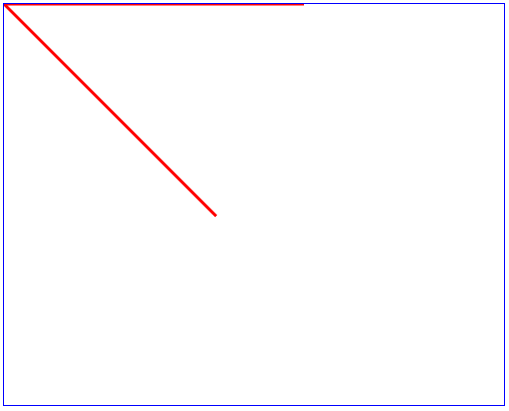.

## 3 - Scaling

We can scale shapes in an HTML 5 canvas using the method scale().

Copy and paste the code below in Notepad++ (or any other HTML5 editor) and run it.

<!DOCTYPE html>
<html>
<body>
<canvas id="myFirstCanvas" width="500" height="401" style="border:1px solid #0000ff;">
This browser does not support the HTML5 canvas tag. </canvas>

<script>

var canvas1 = document.getElementById('myFirstCanvas');
var context1 = canvas1.getContext('2d');

// Draw shape

context1.beginPath();
context1.strokeStyle = "red";
context1.lineWidth = 1;
context1.rect(20,20,100,100);
context1.stroke();

// Scale shape
context1.scale(3,2);

context1.beginPath();
context1.strokeStyle = "green";
context1.lineWidth = 1;
context1.rect(20,20,100,100);
context1.stroke();

</script>
</body>
</html>

Explanations
The method scale() in the statement context1.scale(3,2); is used to scale the width (along x) by 3 and the height (along y) by 2.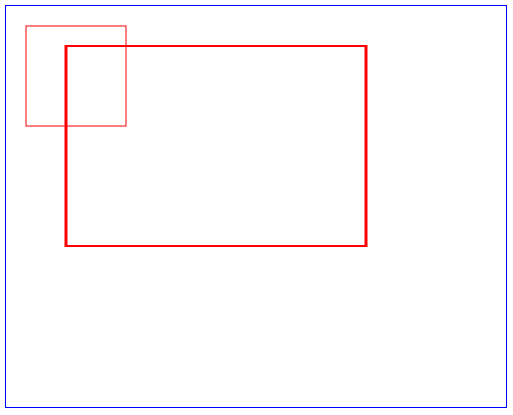.

## 4 - Transformations Accumulate

If several transformations are applied, their effects will accumulate.

Try the example below.

<!DOCTYPE html>
<html>
<body>
<canvas id="myFirstCanvas" width="500" height="401" style="border:1px solid #0000ff;">
This browser does not support the HTML5 canvas tag. </canvas>

<script>

var canvas1 = document.getElementById('myFirstCanvas');
var context1 = canvas1.getContext('2d');

// Draw shape

context1.beginPath();
context1.strokeStyle = "red";
context1.lineWidth = 1;
context1.rect(50,50,80,80);
context1.stroke();

// Scale shape
context1.scale(2,2);

context1.beginPath();
context1.strokeStyle = "green";
context1.lineWidth = 1;
context1.rect(50,50,80,80);
context1.stroke();

// Rotate shape
context1.rotate((20)*Math.PI/180);

context1.beginPath();
context1.strokeStyle = "blue";
context1.lineWidth = 1;
context1.rect(50,50,80,80);
context1.stroke();

</script>
</body>
</html>

Explanations
The rectangle is scaled and then rotated.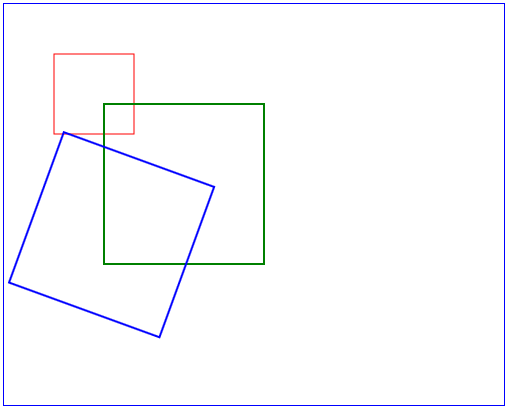.

## 5 - Several Transformations: setTransform()

The setTransform(xScale, xSkew, ySkew, yScale, xTranslate, yTranslate) method allows several transformation with one statement as follows:

xScale : the scaling (increase if greater than 1 or decrease if less than 1) along the x axis.

xSkew : rotation of the x axis by an angle whose tangent is xSkew.

ySkew : rotation of the y axis by an angle whose tangent is ySkew.

yScale : the scaling (increase if greater than 1 or decrease if less than 1) along the y axis.

xTranslate : amount of translation along the x axis

yTranslate : amount of translation along the y axis

Try the example below.
<!DOCTYPE html>
<html>
<body>
<canvas id="myFirstCanvas" width="500" height="401" style="border:1px solid #0000ff;">
This browser does not support the HTML5 canvas tag. </canvas>

<script>
var canvas1 = document.getElementById('myFirstCanvas');
var context1 = canvas1.getContext('2d');

// Draw shape
context1.beginPath();
context1.strokeStyle = "red";
context1.lineWidth = 1;
context1.rect(50,50,80,80);
context1.stroke();

// Draw same shape with translation of 100 along x and 200 along y
context1.setTransform(1,0,0,1,100,200);
context1.beginPath();
context1.strokeStyle = "blue";
context1.lineWidth = 1;
context1.rect(50,50,80,80);
context1.stroke();

// Draw same shape with 3 types of transformations
//(1)scaled by 2 along x
//(2)skewed along x by 0.4 and y by 0.4
//(3)translated by 50 along x and 50 along y
context1.setTransform(2,0.4,0.4,1,50,50);
context1.beginPath();
context1.strokeStyle = "green";
context1.lineWidth = 1;
context1.rect(50,50,80,80);
context1.stroke();

</script>
</body>
</html>

Explanations
The method setTransform(1,0,0,1,100,200) in the statement context1.setTransform(1,0,0,1,100,200); will only translate the rectangle by 100 pixels along x and 200 pixels along the y axis. (blue rectangle)

The method setTransform(2,0.4,0.4,1,50,50) in the statement context1.setTransform(2,0.4,0.4,1,50,50); will scale by 2 along the x axis, and by 1 (no scaling) along the y axis. The rectangle is translated by 50 pixels along the x axis and 50 pixels along the y axis. The rectangle is skewed by angle θ1 = arctan(0.4) = 21.8 degrees with respect to the x axis and an angle θ2 = arctan(0.4) = 21.8 degrees with respect to the y axis.(green parallelogram)

Note that the two sets of transformations do no accumulate.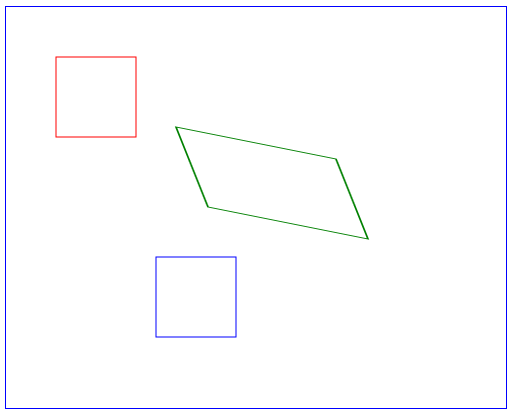.